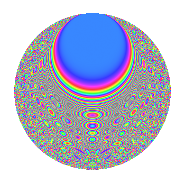# Properties

 Label 2541.2.iLevel 2541 Weight 2 Character orbit i Rep. character $$\chi_{2541}(1453,\cdot)$$ Character field $$\Q(\zeta_{3})$$ Dimension 290 Sturm bound 704

# Related objects

## Defining parameters

 Level: $$N$$ = $$2541 = 3 \cdot 7 \cdot 11^{2}$$ Weight: $$k$$ = $$2$$ Character orbit: $$[\chi]$$ = 2541.i (of order $$3$$ and degree $$2$$) Character conductor: $$\operatorname{cond}(\chi)$$ = $$7$$ Character field: $$\Q(\zeta_{3})$$ Sturm bound: $$704$$

## Dimensions

The following table gives the dimensions of various subspaces of $$M_{2}(2541, [\chi])$$.

Total New Old
Modular forms 752 290 462
Cusp forms 656 290 366
Eisenstein series 96 0 96

## Trace form

 $$290q + 2q^{2} - q^{3} - 142q^{4} - 2q^{5} - 4q^{6} + 3q^{7} - 24q^{8} - 145q^{9} + O(q^{10})$$ $$290q + 2q^{2} - q^{3} - 142q^{4} - 2q^{5} - 4q^{6} + 3q^{7} - 24q^{8} - 145q^{9} + 8q^{10} - 2q^{12} - 14q^{13} - 14q^{14} + 4q^{15} - 144q^{16} + 2q^{18} + 7q^{19} + 56q^{20} + 4q^{21} - 8q^{23} - 151q^{25} + 22q^{26} + 2q^{27} - 8q^{29} + 12q^{30} - 13q^{31} + 36q^{32} - 46q^{35} + 284q^{36} + q^{37} - 10q^{38} - q^{39} + 12q^{40} + 52q^{41} - 10q^{42} + 2q^{43} - 2q^{45} + 32q^{46} + 18q^{47} - 8q^{48} - 37q^{49} - 60q^{50} - 8q^{51} + 10q^{52} - 28q^{53} + 2q^{54} - 24q^{56} - 14q^{57} + 12q^{58} + 12q^{59} + 16q^{60} + 2q^{61} + 52q^{62} - 3q^{63} + 256q^{64} - 42q^{65} + 5q^{67} - 36q^{68} + 32q^{69} - 16q^{70} + 28q^{71} + 12q^{72} + 27q^{73} - 42q^{74} - 15q^{75} - 124q^{76} - 20q^{78} - 15q^{79} + 8q^{80} - 145q^{81} - 20q^{82} + 20q^{83} + 50q^{84} - 8q^{85} + 38q^{86} + 16q^{87} + 32q^{89} - 16q^{90} + 111q^{91} - 80q^{92} + 43q^{93} + 40q^{94} - 18q^{95} + 12q^{96} + 76q^{97} - 16q^{98} + O(q^{100})$$

## Decomposition of $$S_{2}^{\mathrm{new}}(2541, [\chi])$$ into newform subspaces

The newforms in this space have not yet been added to the LMFDB.

## Decomposition of $$S_{2}^{\mathrm{old}}(2541, [\chi])$$ into lower level spaces

$$S_{2}^{\mathrm{old}}(2541, [\chi]) \cong$$ $$S_{2}^{\mathrm{new}}(21, [\chi])$$$$^{\oplus 3}$$$$\oplus$$$$S_{2}^{\mathrm{new}}(77, [\chi])$$$$^{\oplus 4}$$$$\oplus$$$$S_{2}^{\mathrm{new}}(231, [\chi])$$$$^{\oplus 2}$$$$\oplus$$$$S_{2}^{\mathrm{new}}(847, [\chi])$$$$^{\oplus 2}$$

## Hecke Characteristic Polynomials

There are no characteristic polynomials of Hecke operators in the database